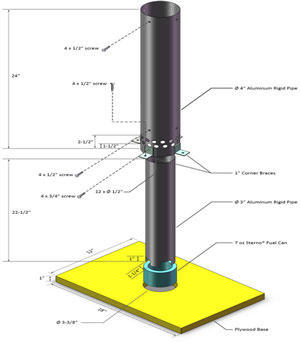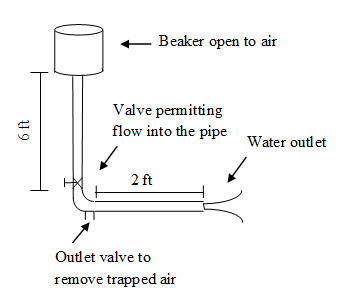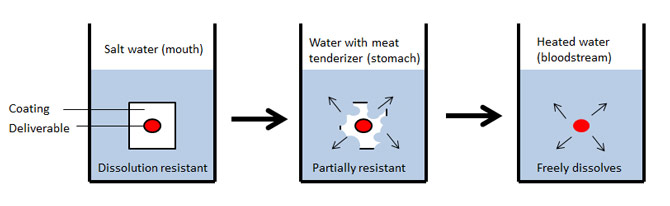# STEM Modules

The following STEM modules are currently in development. All will be free to use but may have some initial cost in terms of supplies - all of which you should be able to obtain in your region:

HOT AIR BALLOONS: (cost = ~\$20)
Subject matter: ideal gas law, density, temperature
Fields of interest: physics, engineeringDRAG REDUCING POLYMERS: (cost = ~\$10)
Subject matter: friction, fluid
Fields of interest: physics, chemistry, engineeringDRUG DELIVERY: (cost = ~\$10)
Subject matter: medicine, human physiology and anatomy
Fields of interest: biology, chemistry, engineeringSUPERBALLS: (cost = ~\$10)
Subject matter: area, mass, synthesis, concentration, elasticity
Fields of interest: math, physics, chemistry, engineering

DRAG REDUCING POLYMERS: (cost = ~\$10)
Subject matter: friction, fluid
Fields of interest: physics, chemistry, engineering